### Explore..Discover..Learn

Explore data using a simple interface. Generate descriptive statistics, understand distributions, build visualizations step by step, perform parametric/non-paramaetric tests and customer segmentation, and build linear and logistic regression models.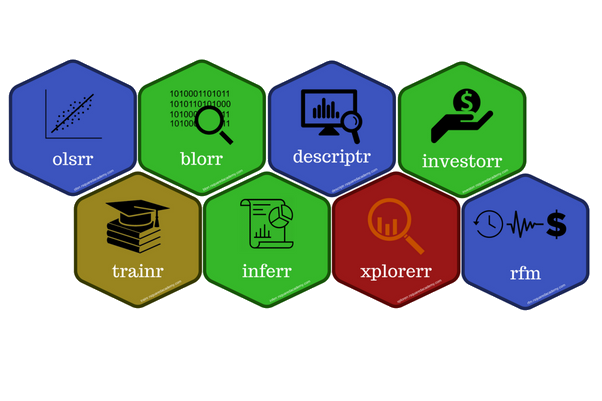### Accelerate your learning with our interactive apps.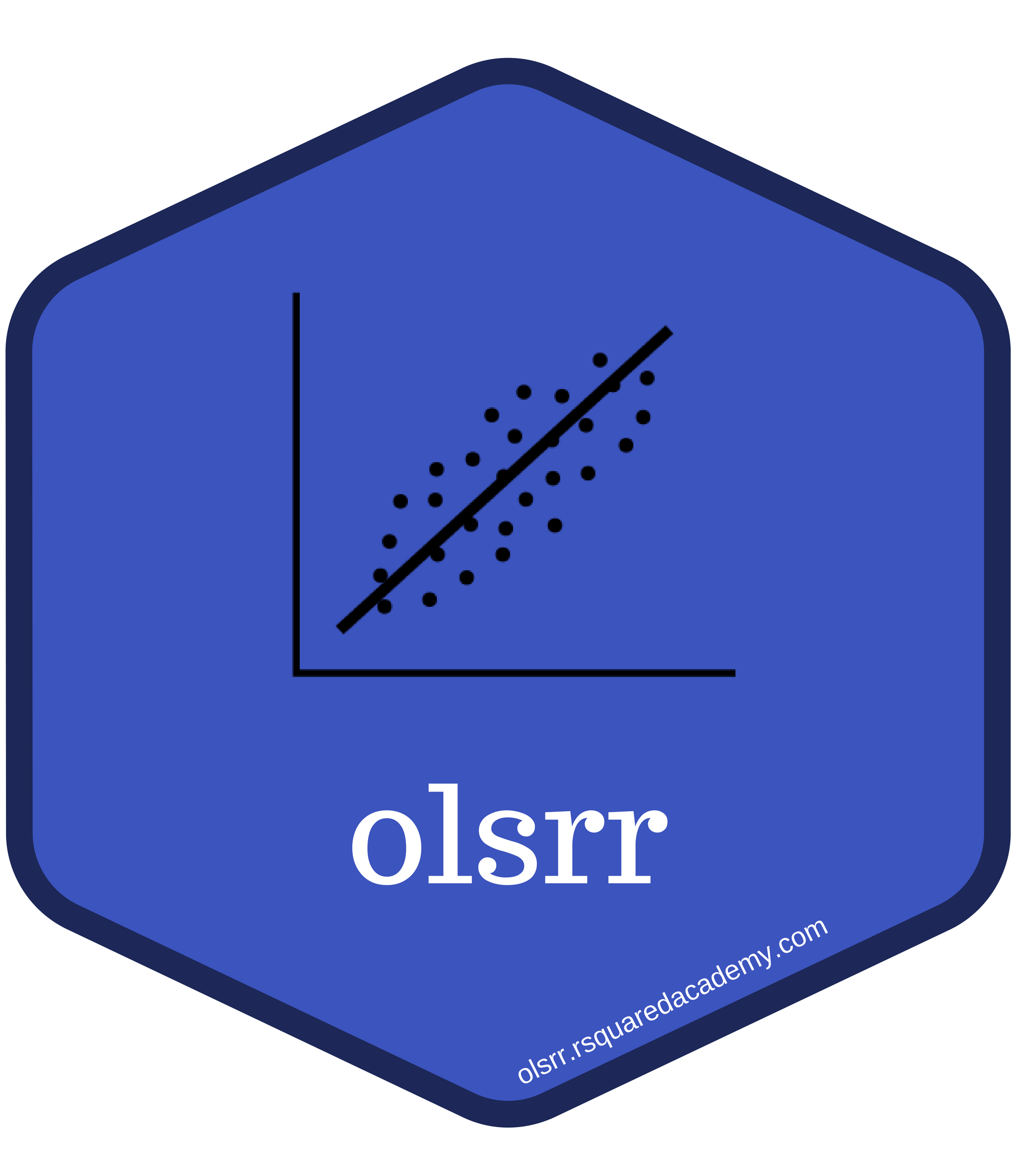##### olsrr

Build linear regression models using the olsrr package. Quickly iterate through models and generate models with the click of a button.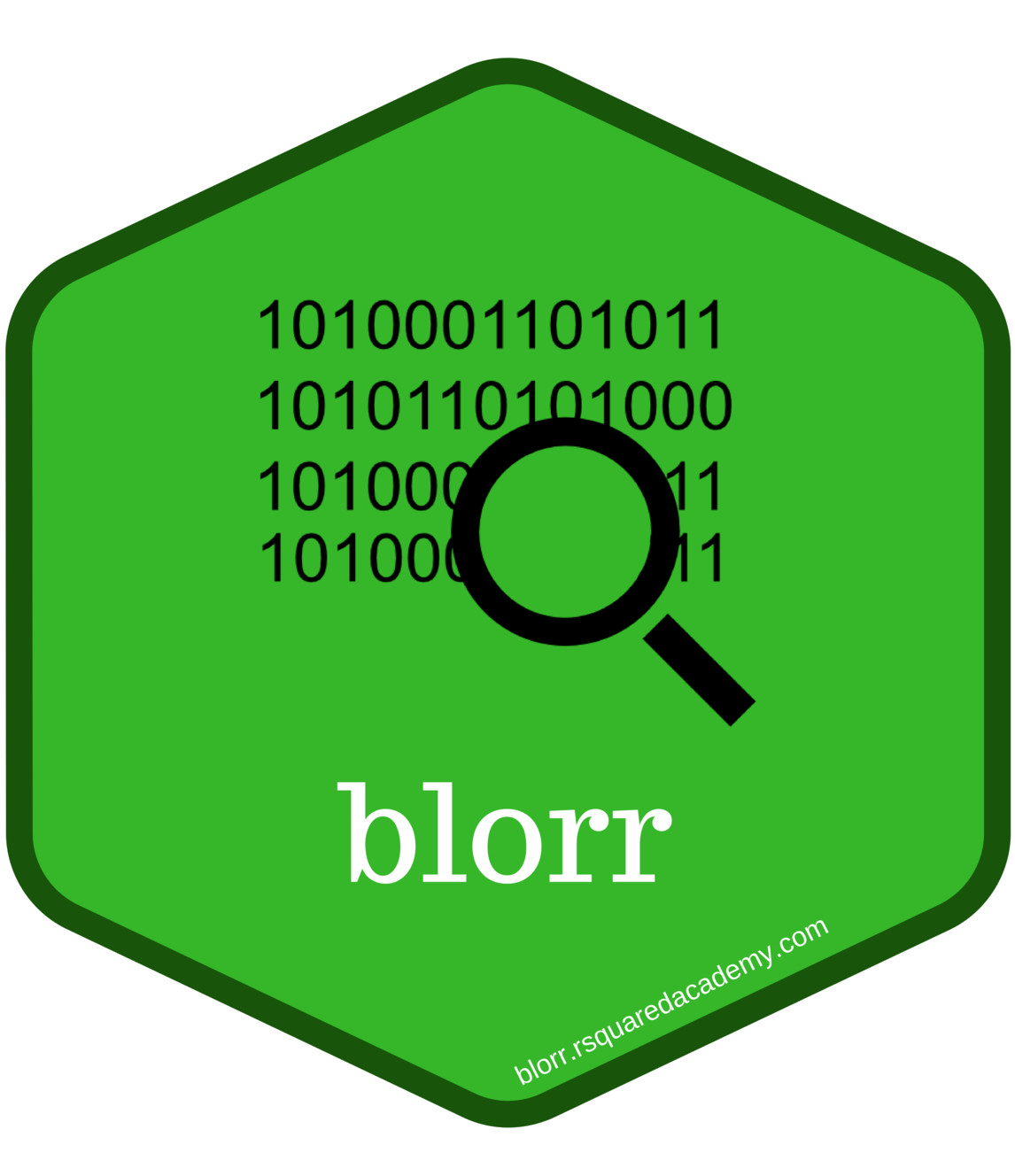##### blorr

Build, validate and monitor binary logistic regression models. Includes comprehensive regression output, model fit and validation statistics.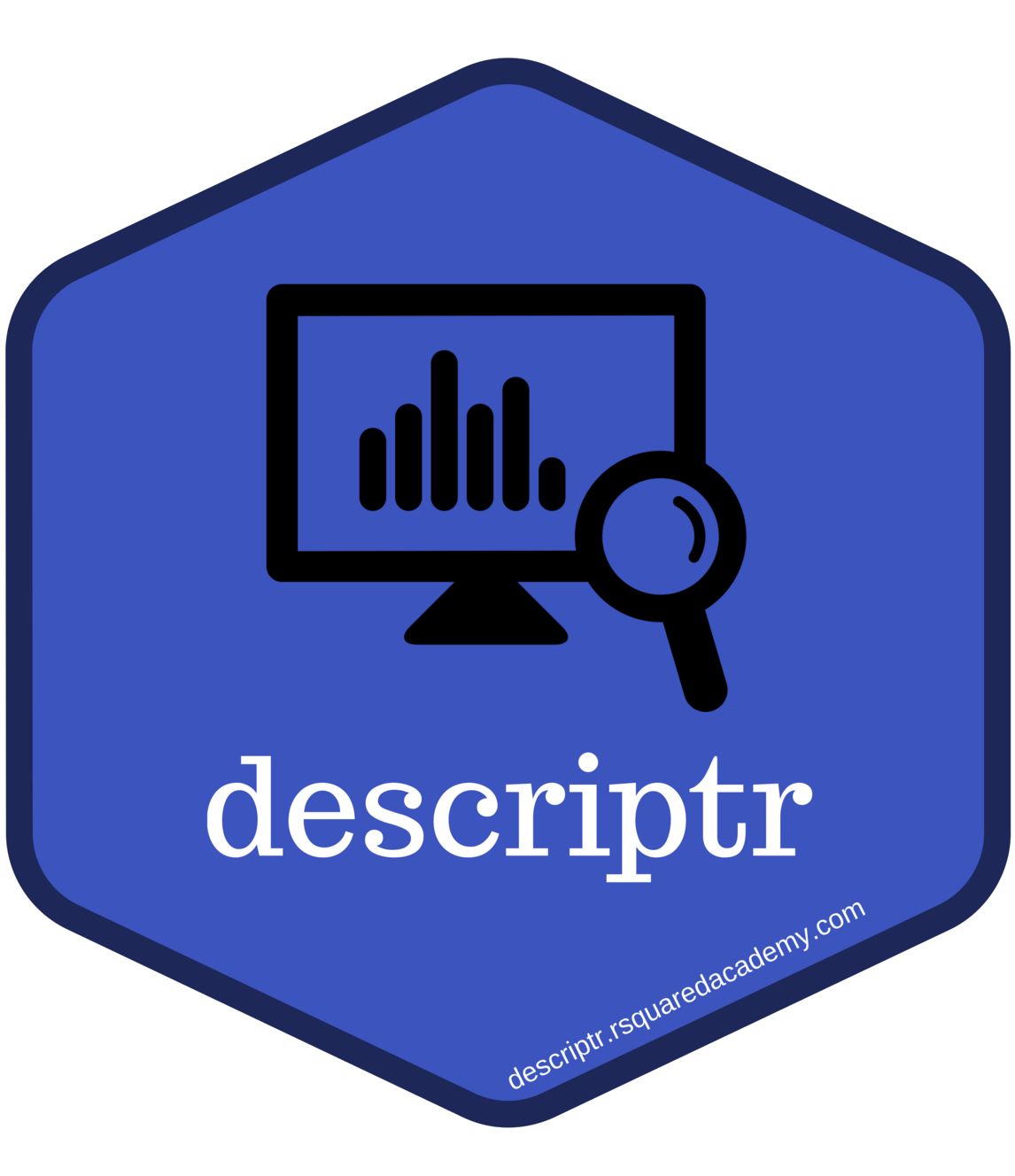##### descriptr

Generate and visualize descriptive statistics for numeric/categorical data.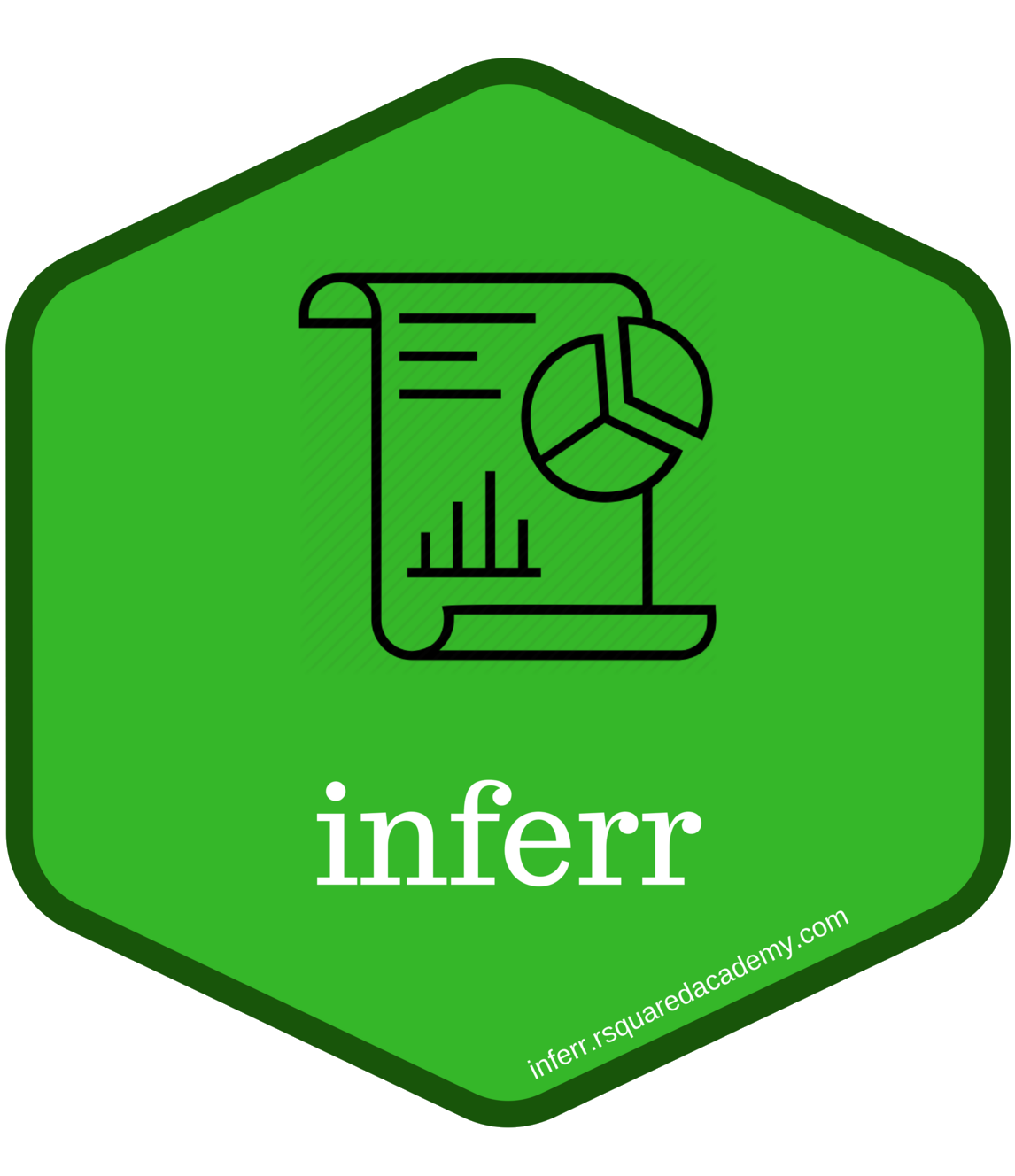##### inferr

Beginner friendly interface to perform hypothesis testing using parametric and non-parametric statistical test. Includes flexible input options and structured results.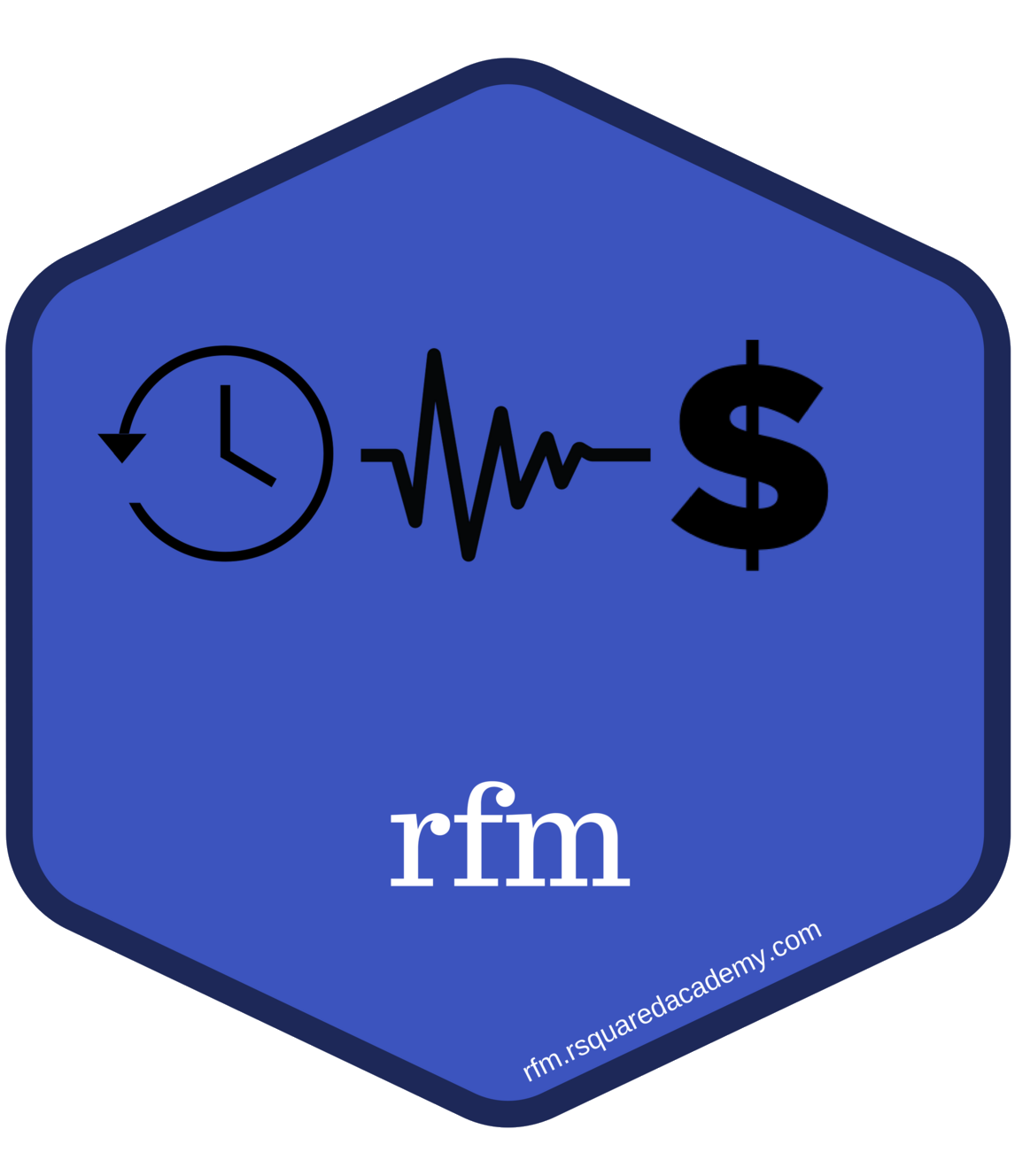##### rfm

Tools for customer segmentation using recency, frequency and monetary value analysis. Build customized segments using customer or transaction level data.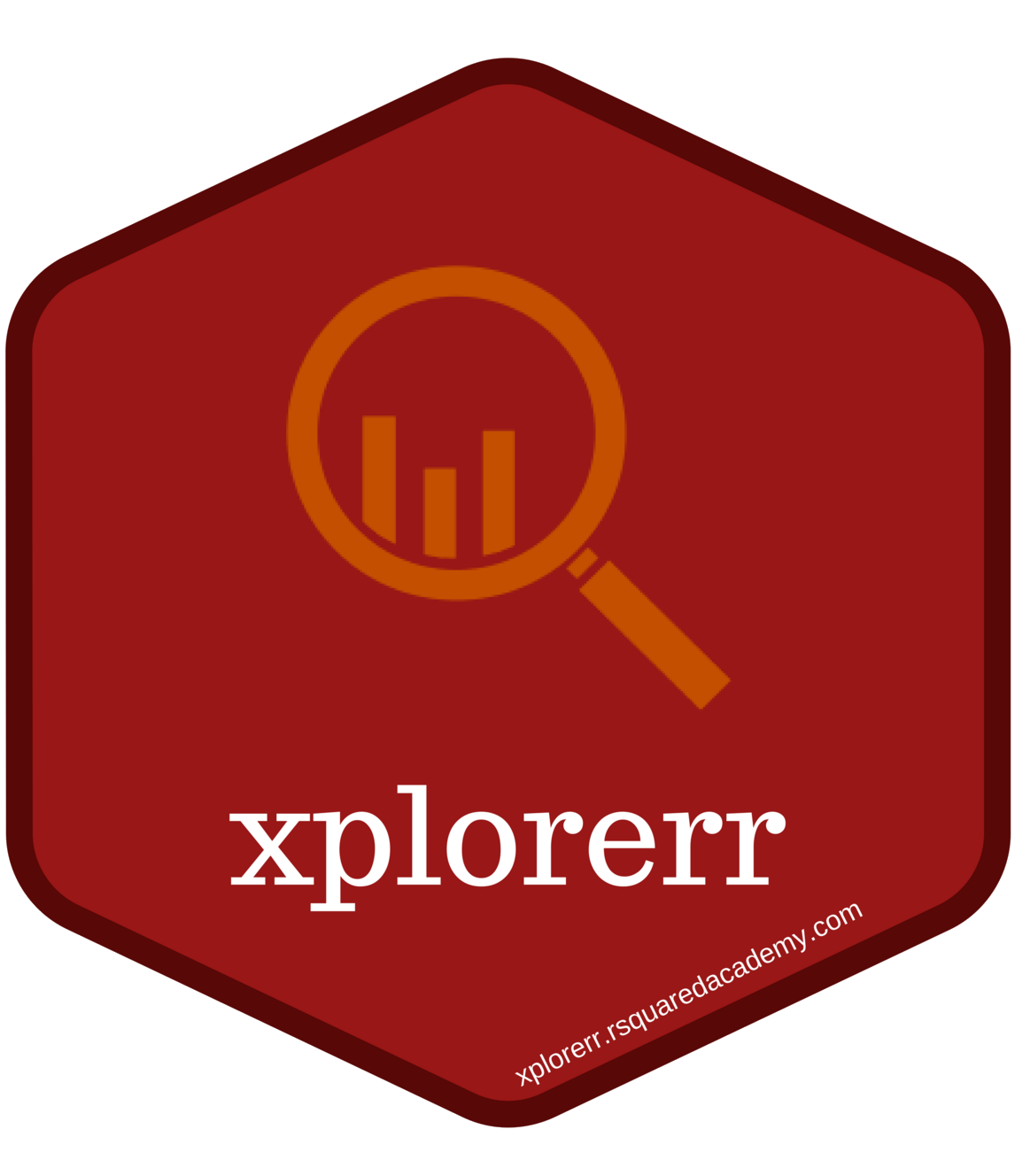##### xplorerr

Apps for data visualization, exploratory data analysis, hypothesis testing, customer segmentation, linear and logistic regression modeling.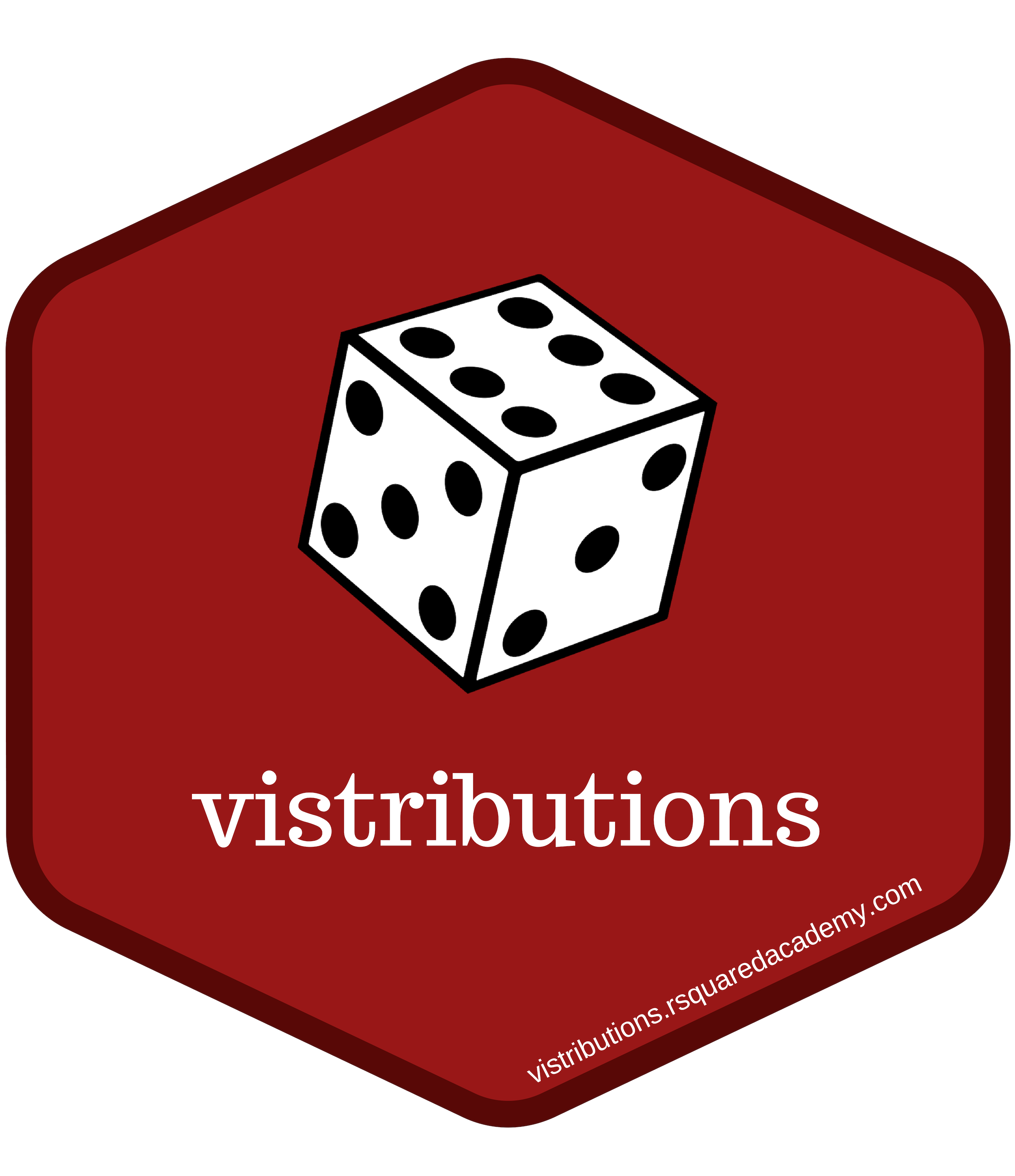##### vistributions

Visualize probability distributions.

## Simple Interface

Simple interface for uploading data. As a tool aimed at beginners, all you need to do is to upload your data in a comma or tab separated file and get going with your analysis.

## Transform Data

Screen data for missing values, rename variables and modify data types.

## Exploratory Data Analysis

Generate detailed summary statistics, one and two way tables, group summaries and visualize the same.

## Understand Statistical Distributions

Vizually explore the most important statistical distributions. Understand the factors which influence their shapes. Learn to calculate the probability and quantiles.

## Visualize Data

Visualize data by interactively building plots step by step.

## Inferential Statistics

Test hypothesis using a set of most important parametric and non-parametric tests.

## Model Building & Validation

Build and evaluate statistical models using a simple workflow.